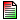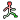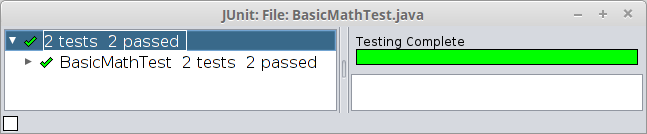```/**
* CS139 - Programming Fundamentals
* Department of Computer Science
* @version Spring 2016
*/
```

# Program testing with JUnit

## Background

One thing we've learned through decades of software engineering is that developing correct software is difficult! The best way to ensure correctness is to test thoroughly while software is being developed. JUnit is a framework that automates testing by providing a standard format for tests and an easy way to execute them (see JUnit FAQ). In today's lab, you will design and implement your own JUnit tests cases.

Collaboration: You are encouraged to work with another student to complete this lab.

## Objectives

• Create a test class for use with JUnit.

• Write JUnit test methods that use `assertEquals`.

• Run JUnit tests for a class or set of classes.

## Key Terms

test class
A class that has the purpose of testing some other class, but is not part of the final application.
test method
A method that has the purpose of testing another method, but is not part of the final application.
assertion
A statement that should always be true. Assertions make claims about the expecte behavior of programs.

## Part 0: Preparation

Watch this video on unit testing before proceeding with the rest of the lab. (Sorry about the sound quality.)

## Part 1: Using JUnit for Testing

The basic JUnit pattern is simple:

• For every class `X` there is a companion class named `XTest` that is responsible for testing the class.
• For every method `m` there is at least one companion method `testM` that is responsible for testing the method.
Class Being Developed Test Class
```public class BasicMath {

public static double add(double x, double y) {
double sum;
sum = x + y;
return sum;
}

public static double subtract(double x, double y) {
double diff;
diff = x - y;
return diff;
}

}
```
```import org.junit.Assert;
import static org.junit.Assert.*;
import org.junit.Before;
import org.junit.Test;

public class BasicMathTest {

double expected;
double actual;

expected = 15.5;
assertEquals(expected, actual, .00001);
}

@Test public void testSubtract() {
double expected;
double actual;

expected = 2.2;
actual = BasicMath.subtract(3.5, 1.3);
assertEquals(expected, actual, .00001);
}

}
```

Note in the above example:

• Each test is preceded by `@Test`. This is referred to as an annotation. In this case, the `@Test` annotation tells JUnit to treat the labeled method as a test method.
• Test methods are not `static`. They are void and take no parameters. However, they can be as complex as you like.
• Each test method establishes an expected value and runs the corresponding method to get the actual value.
• Finally, test methods use `assertEquals` (or other assert methods provided by JUnit) to verify correctness.
• Normally, `assertEquals` takes two arguments: the first is the expected result, the second is the actual result. When `assertEquals` is used on floating point results, it takes a third argument that specifies an acceptable degree of error. The test above will pass as long as the actual value is within .00001 of the expected value. (It usually doesn't make sense to test floating point values for exact equality. We want to ignore tiny rounding errors.)

## Configuring jGRASP to use JUnit

2. Start jGRASP and select "Tools -> JUnit -> Configure". For "JUnit Home" select the directory where you stored the two .jar files.

If all went well the "Create JUnit test file" buttonshould now appear whenever you open a Java file in jGRASP. Clicking that button will auto-generate a starter test class with the appropriate imports.

## Running JUnit in jGRASP

• Create a new Java file in jGRASP using the example code from BasicMath.java above. Save the file.
• Click the "Create JUnit test file" button. You should see a new file that looks like the following.
```import org.junit.Assert;
import static org.junit.Assert.*;
import org.junit.Before;
import org.junit.Test;

public class BasicMathTest {

/** Fixture initialization (common initialization
*  for all tests). **/
@Before public void setUp() {
}

/** A test that always fails. **/
@Test public void defaultTest() {
Assert.assertEquals("Default test added by jGRASP. Delete "
}
}
```
• Delete the two auto-generated methods, and replace them with the testing methods provided above.
• Press thebutton to compile and run the JUnit tests. You should see a window like the following indicating that both tests have passed.• Now go back and "break" the code by changing the `BasicMath.add` method: `return sum + 1;`

• Compile the new code, and then press thebutton. What happens when an assertion fails?

## Part 2: Writing Basic Test Methods

• Implement the following methods in your BasicMath class:

• public static double multiply(double x, double y)
• public static double divide(double x, double y)
• Now write the corresponding test methods in BasicMathTest. Use the same pattern as in testAdd and testSubtract: establish an expected result and an actual result, then compare the two with an assertion.

• Run your new tests to validate the new methods. Then introduce errors into the new methods, just as you did before with the add method, and run the tests again to see if they are really working.

It's generally not enough to test a method just once. To be sure that the method is correct, we need to test multiple times with multiple values.

• Add the following test cases to the testAdd method. (Copy and paste the last three lines of testAdd for each case below. For readability, separate each one with a blank line.)

• case 1: x = 0.0, y = 0.0
• case 2: x = -5.0, y = 3.5
• Add two additional test cases to each of the other three test methods. Determine your own expected values for these methods.

• Write an appropriate Javadoc comment for your BasicMathTest class, including `@author` and `@version` tags.

• Create a .zip file containing both both BasicMath.java and BasicMathTest.java.

This can be done on the lab computers by selecting both files in the file browser, right clicking and selecting "Compress...". Choose ".zip" from the pull-down menu. Name the file something reasonable like BasicMath.zip or JUnitLab.zip.

• Submit your .zip file through Web-CAT.

Web-CAT will evaluate both files: it will evaluate BasicMath.java on the basis of correctness, and it will evaluate BasicMathTest.java on the basis of coverage. Your submission will only get full credit if every method in BasicMath.java is covered by one of your tests in BasicMathTest.java.

Web-CAT will not run Checkstyle on your submission.

## Part 3: A More Complex Example (If Time)

Add the following code to the end of your BasicMath class, but don't look too carefully at the code itself. Your goal is to find the mistakes in calculateTax by writing test cases based on its documentation.

```    /**
* Calculate the tax on the given amount based on the following rules:
*   If the taxType is 'X' or 'x' (exempt), then tax amount is zero.
*   If the taxType is 'M' or 'm', then tax is 11% of the amount.
*   If the taxType is 'F' or 'f', then tax is 2% of the amount.
*   If the taxType is anything else, then tax is 5% of the amount.
*
* @param amount the amount of the sale
* @param taxType type of items purchased
* @return amount of tax
*/
public static double calculateTax(double amount, char taxType) {
double tax;
switch (taxType) {
case 'X':
case 'x':
tax = 0.0;
case 'M':
case 'n':
tax = 0.11 * amount;
case 'F':
case 'f':
tax = 0.2 * amount;
default:
tax = 0.5 * amount;
}
return tax;
}
```
1. Rather than write over a dozen test cases in a single method, create the following test methods:

• testTypeX should test cases 'X' and 'x'
• testTypeM should test cases 'M' and 'm'
• testTypeF should test cases 'F' and 'f'
• testOther should test "anything else"
2. For each of the calculateTax test methods, write at least four test cases using assertEquals. Note that you can implement many of these assertions in one line of code, for example:

`assertEquals(0.0, BasicMath.calculateTax(1.99, 'X'));`
3. Run your test cases. If all your test methods are correct, all four of them should fail.

4. OPTIONAL: Can you figure out how to fix calculateTax and get your test methods to pass? You may need to learn about The switch Statement in the Java tutorials.